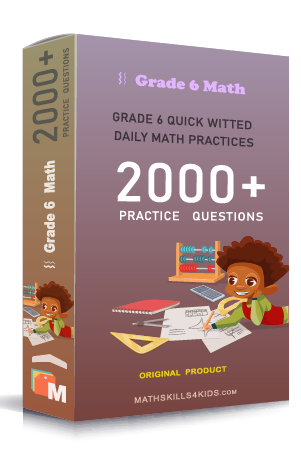# Grade 6 Mixed operations with solutions

Subject
Math

Resource Type
Worksheets, Printables, Homeschool

Standards
CCSS 6.NS.B.3 | CCSS 6.EE.A.2c

Format
PDF (15.5 MB | 22 pages → Exercises + Solutions)

• ### -15% OFF Over \$100

Promo Code: TOPLA

After Purchase

\$2.85

This package allows you to practice the following skills

Add subtract multiply or divide two whole numbers

Add subtract multiply or divide two whole numbers word problems

Evaluate numerical expressions involving whole numbers

Add subtract multiply or divide two decimals

Add subtract multiply or divide two decimals word problems

Evaluate numerical expressions involving decimals

Add subtract multiply or divide two fractions

Add subtract multiply or divide two fractions word problems

Evaluate numerical expressions involving fractions

Add subtract multiply or divide two integers

Evaluate numerical expressions involving integers

This product is a part of the Mega Pack
* Grade 6 quick-witted daily math practices *